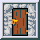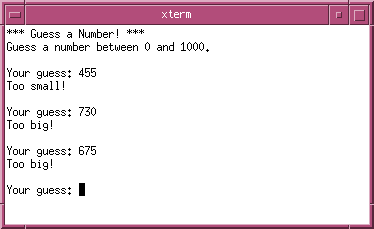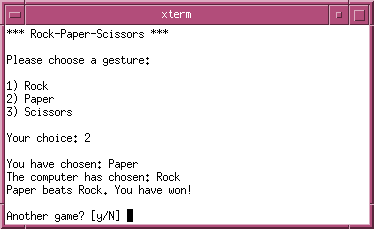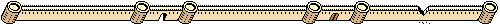# Simple Games in Python

A collection of simple games, written in Python 3:

The source code of all games is published as public domain.

## Guess a Number!Try to guess a random number between 0 and 1000 the computer has chosen.

### Source Code

``````#!/usr/bin/env python3

import random

random.seed()

secret = random.randint(1, 1000)

guess = 0
attempts = 0

print('*** Guess a Number! ***')
print('Guess a number between 0 and 1000.')

while guess != secret:
guess = int(input('\nYour guess: '))

if guess < secret:
print('Too small!')

if guess > secret:
print('Too big!')

attempts = attempts + 1

print('\n{} is correct!'.format(guess))
print('You needed {} attempts.'.format(attempts))``````

## Rock-Paper-ScissorsA simple game played between two people, in which each of them has to form one of three gestures: 👊 rock, ✋ paper, or ✌ scissors. In this version, the player has to compete against the computer.

### Source Code

``````#!/usr/bin/env python3

import random

random.seed()

symbols = { 1 : 'Rock', 2 : 'Paper', 3 : 'Scissors' }
rules = { 1 : 3, 2 : 1, 3 : 2 }
done = False

print('*** Rock-Paper-Scissors ***')

while not done:
# player's choice
print('\nPlease choose a gesture:\n')
print('1) Rock')
print('2) Paper')
print('3) Scissors')

try:
player = int(input('\nYour choice: '))
except ValueError:
print('Invalid input.')
continue

print('\nYou have chosen: {}'.format(symbols[player]))

# computer's choice
computer = random.randint(1, 3)
print('The computer has chosen: {}'.format(symbols[computer]))

# results
if player == computer:
print('Draw!')
elif rules[player] == computer:
print('{} beats {}. You have won!'
.format(symbols[player], symbols[computer]))
else:
print('{} beats {}. The computer has won!'
.format(symbols[computer], symbols[player]))

# replay or quit
replay = input('\nAnother game? [y/N] ')

if replay.lower() != 'y':
done = True``````

## Quiz

comming soon …Last updated: Sun, 10 Feb 2019 18:52:32 +0100